F Statistics

Suppose that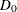is the deviance resulting from fitting a generalized linear model and that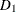is the deviance from fitting a submodel. Then, under appropriate regularity conditions, the asymptotic distribution of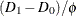is chi-square with r degrees of freedom, where r is the difference in the number of parameters between the two models andis the dispersion parameter. Ifis unknown, andis an estimate ofbased on the deviance or Pearson’s chi-square divided by degrees of freedom, then, under regularity conditions,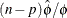has an asymptotic chi-square distribution with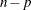degrees of freedom. Here, n is the number of observations and p is the number of parameters in the model that is used to estimate. Thus, the asymptotic distribution of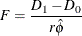is the F distribution with r anddegrees of freedom, assuming thatandare approximately independent.

This F statistic is computed for the Type 1 analysis, Type 3 analysis, and hypothesis tests specified in CONTRAST statements when the dispersion parameter is estimated by either the deviance or Pearson’s chi-square divided by degrees of freedom, as specified by the DSCALE or PSCALE option in the MODEL statement. In the case of a Type 1 analysis, model 0 is the higher-order model obtained by including one additional effect in model 1. For a Type 3 analysis and hypothesis tests, model 0 is the full specified model and model 1 is the submodel obtained from constraining the Type III contrast or the user-specified contrast to be 0.